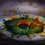# Polynomial Expansions (useful formulas)

Let $\mathrm{A}$ be the set of all "$\color{#3D99F6}{\text{useful}}$" things.

Let $\mathrm{B}$ be the set of all "$\color{#20A900}{\text{Awesome}}$" things.

Let $\mathrm{C}$ be the set of all "$\color{#D61F06}{\text{fascinating}}$" things.

Let $\mathrm{D}$ be the set of all "$\color{#624F41}{\text{easily understandable}}$" things.

What this note contains is an element of $\mathrm{A \cap B \cap C \cap D}$....

$\color{#3D99F6}{\textbf{Polynomial Expansions}}$

$\mathbf{1.}\quad \displaystyle \dfrac{1-x^{m+1}}{1-x} = 1+x+x^2+...+x^m = \sum_{k=0}^m x^k$

$\mathbf{2.} \quad \displaystyle \dfrac{1}{1-x} = 1+x+x^2+... = \sum_{k=0}^\infty x^k$

$\mathbf{3.}\quad \displaystyle (1+x)^n = 1+\binom{n}{1} x + \binom{n}{2}x^2+...+\binom{n}{n}x^n = \sum_{k=0}^n \dbinom{n}{k} x^k$

$\mathbf{4.}\quad \displaystyle (1-x^m)^n = 1-\binom{n}{1}x^m+\binom{n}{2}x^{2m}-...+(-1)^n\binom{n}{n} x^{nm}$

$\quad \quad \quad \quad\quad \displaystyle = \sum_{k=0}^n (-1)^n \dbinom{n}{k}x^{km}$

$\mathbf{5.}\quad \displaystyle\dfrac{1}{(1-x)^n} = 1+ \binom{1+n-1}{1} x + \binom{2+n-1}{2} x^2+...+\binom{r+n-1}{r} x^r+......$

$\quad \quad \quad \quad \quad \displaystyle =\sum_{k=0}^\infty \dbinom{k+n-1}{k} x^k$

$\color{#69047E}{\text{Tremendously useful}}$ in calculating the $\color{#3D99F6}{\text{co-efficient}}$ of any term in specially generating functions that we come across, in many combinatorics problems...

Taken From - Alan Tucker's "Applied Combinatorics"

Good luck problem solving !7 years ago

This discussion board is a place to discuss our Daily Challenges and the math and science related to those challenges. Explanations are more than just a solution — they should explain the steps and thinking strategies that you used to obtain the solution. Comments should further the discussion of math and science.

When posting on Brilliant:

• Use the emojis to react to an explanation, whether you're congratulating a job well done , or just really confused .
• Ask specific questions about the challenge or the steps in somebody's explanation. Well-posed questions can add a lot to the discussion, but posting "I don't understand!" doesn't help anyone.
• Try to contribute something new to the discussion, whether it is an extension, generalization or other idea related to the challenge.

MarkdownAppears as
*italics* or _italics_ italics
**bold** or __bold__ bold
- bulleted- list
• bulleted
• list
1. numbered2. list
1. numbered
2. list
Note: you must add a full line of space before and after lists for them to show up correctly
paragraph 1paragraph 2

paragraph 1

paragraph 2

[example link](https://brilliant.org)example link
> This is a quote
This is a quote
    # I indented these lines
# 4 spaces, and now they show
# up as a code block.

print "hello world"
# I indented these lines
# 4 spaces, and now they show
# up as a code block.

print "hello world"
MathAppears as
Remember to wrap math in $$ ... $$ or $ ... $ to ensure proper formatting.
2 \times 3 $2 \times 3$
2^{34} $2^{34}$
a_{i-1} $a_{i-1}$
\frac{2}{3} $\frac{2}{3}$
\sqrt{2} $\sqrt{2}$
\sum_{i=1}^3 $\sum_{i=1}^3$
\sin \theta $\sin \theta$
\boxed{123} $\boxed{123}$

Sort by:

I know that the second one only works for $x<1$. But do any of the others work for only $x>1$. Also, thank you so much for this note, it's very useful.

- 7 years ago

-1<x<1 actually.

- 6 years, 12 months ago

Thank you, but do u know which ones only work for this case

- 6 years, 12 months ago

Can you add parts of this page into the algebra wiki? I think that Algebraic Identities and Algebraic Manipulation - Identities, would be suitable places to add them.

Staff - 6 years, 9 months ago

what is the derivation of 5th one.

- 7 years ago

Standard result, it's related to "Newton's generalised Binomial theorem", but if you do want the derivation please see it here

- 7 years ago

Definitely 2.

- 7 years ago

Does the last one work for 0<x<1???

- 6 years, 11 months ago

set contains Everything

- 6 years, 12 months ago

Is this for nerds like you?

- 6 years, 12 months ago

Dude don't use nerds in a derogatory manner please. If you don't like nerds or aren't one yourself, you should remove yourself from Brilliant.org. Have a nice day.

- 6 years, 11 months ago

@Finn Hulse , thanks for helping here, really !img

I truly like your comment, by these many likes :-img

- 6 years, 11 months ago

Haha, anytime dude. :D

- 6 years, 11 months ago

Agreed. BTW, some nerds can be good at sports as well.

- 6 years, 11 months ago

# (#Sharky_Surprises )

- 6 years, 11 months ago

BTW sharky, participate in JOMO 8, we miss your submission.

@Sharky Kesa , JOMO 8 starts $\color{#D61F06}{\textbf{TOMORROW}}$ and has some good questions I made.

- 6 years, 11 months ago

This is for using in generating functions we design for combinatorics problems.... For example, see the set "vegetable combinatorics".... (type in search bar simply).... That's for all who want to learn, nothing high-figh technique or anything, just formulas to get co-efficient of a specific term in a generating function. @Jack Daniel Zuñiga Cariño

- 6 years, 12 months ago